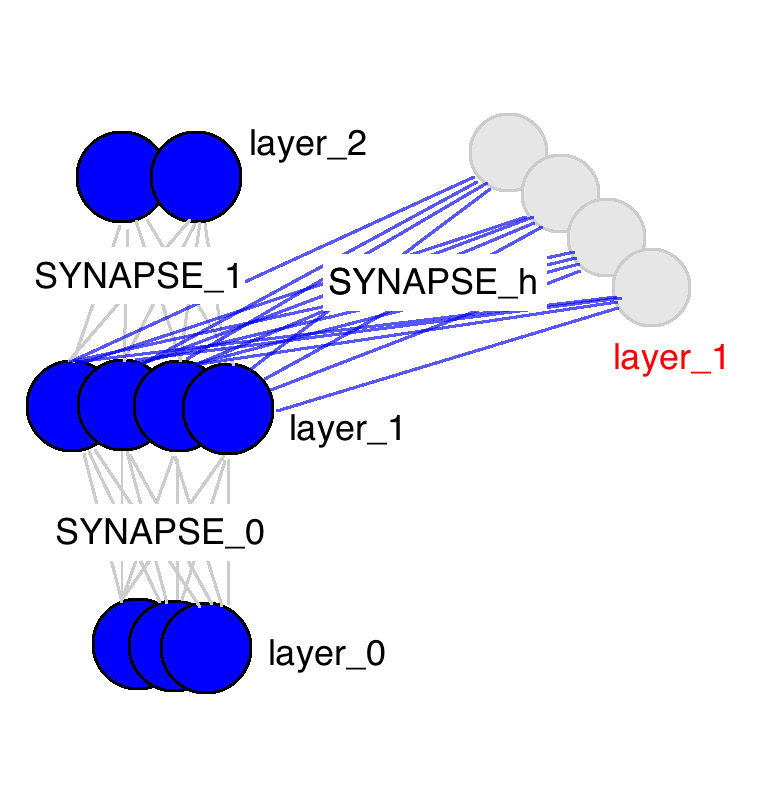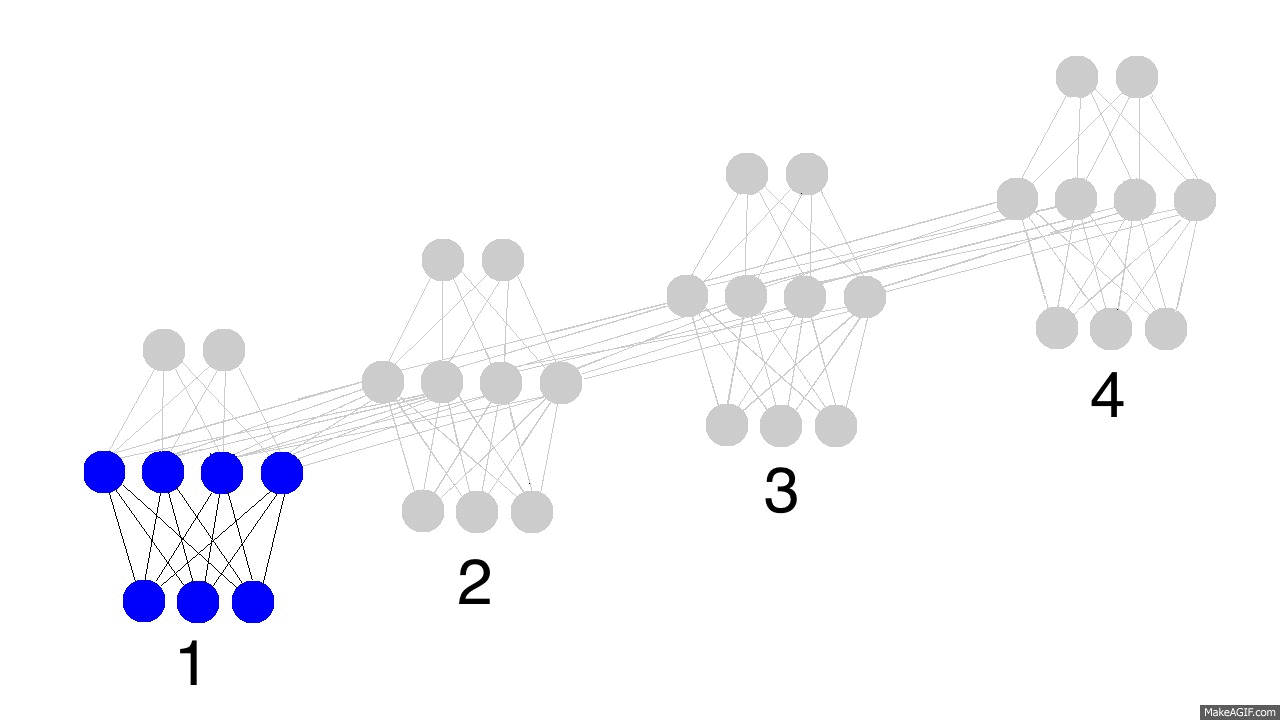废话少说， 给我看看代码

import copy, numpy as np
np.random.seed(0) #固定随机数生成器的种子，便于得到固定的输出，【译者注：完全是为了方便调试用的]

# compute sigmoid nonlinearity
def sigmoid(x): #激活函数
output = 1/(1+np.exp(-x))
return output

# convert output of sigmoid function to its derivative
def sigmoid_output_to_derivative(output):#激活函数的导数
return output*(1-output)

# training dataset generation
int2binary = {} #整数到其二进制表示的映射
binary_dim = 8 #暂时制作256以内的加法， 可以调大

## 以下5行代码计算0-256的二进制表示
largest_number = pow(2,binary_dim)
binary = np.unpackbits(
np.array([range(largest_number)],dtype=np.uint8).T,axis=1)
for i in range(largest_number):
int2binary[i] = binary[i]

# input variables
alpha = 0.1 #学习速率
input_dim = 2 #因为我们是做两个数相加，每次会喂给神经网络两个bit，所以输入的维度是2
hidden_dim = 16 #隐藏层的神经元节点数，远比理论值要大（译者注：理论上而言，应该一个节点就可以记住有无进位了，但我试了发现4的时候都没法收敛），你可以自己调整这个数，看看调大了是容易更快地收敛还是更慢
output_dim = 1 #我们的输出是一个数，所以维度为1

# initialize neural network weights
synapse_0 = 2*np.random.random((input_dim,hidden_dim)) - 1 #输入层到隐藏层的转化矩阵，维度为2*16， 2是输入维度，16是隐藏层维度
synapse_1 = 2*np.random.random((hidden_dim,output_dim)) - 1
synapse_h = 2*np.random.random((hidden_dim,hidden_dim)) - 1
# 译者注：np.random.random产生的是[0,1)的随机数，2 * [0, 1) - 1 => [-1, 1)，
# 是为了有正有负更快地收敛，这涉及到如何初始化参数的问题，通常来说都是靠“经验”或者说“启发式规则”，说得直白一点就是“蒙的”！机器学习里面，超参数的选择，大部分都是这种情况，哈哈。。。
# 我自己试了一下用【0, 2)之间的随机数，貌似不能收敛，用[0,1)就可以，呵呵。。。

# 以下三个分别对应三个矩阵的变化
synapse_0_update = np.zeros_like(synapse_0)
synapse_1_update = np.zeros_like(synapse_1)
synapse_h_update = np.zeros_like(synapse_h)

# training logic
# 学习10000个例子
for j in range(100000):

# 下面6行代码，随机产生两个0-128的数字，并查出他们的二进制表示。为了避免相加之和超过256，这里选择两个0-128的数字
# generate a simple addition problem (a + b = c)
a_int = np.random.randint(largest_number/2) # int version
a = int2binary[a_int] # binary encoding

b_int = np.random.randint(largest_number/2) # int version
b = int2binary[b_int] # binary encoding

c_int = a_int + b_int
c = int2binary[c_int]

# where we'll store our best guess (binary encoded)
# 存储神经网络的预测值
d = np.zeros_like(c)

overallError = 0 #每次把总误差清零

layer_2_deltas = list() #存储每个时间点输出层的误差
layer_1_values = list() #存储每个时间点隐藏层的值
layer_1_values.append(np.zeros(hidden_dim)) #一开始没有隐藏层，所以里面都是0

# moving along the positions in the binary encoding
for position in range(binary_dim):#循环遍历每一个二进制位

# generate input and output
X = np.array([[a[binary_dim - position - 1],b[binary_dim - position - 1]]])#从右到左，每次去两个输入数字的一个bit位
y = np.array([[c[binary_dim - position - 1]]]).T#正确答案

# hidden layer (input ~+ prev_hidden)
layer_1 = sigmoid(np.dot(X,synapse_0) + np.dot(layer_1_values[-1],synapse_h))#（输入层 + 之前的隐藏层） -> 新的隐藏层，这是体现循环神经网络的最核心的地方！！！

# output layer (new binary representation)
layer_2 = sigmoid(np.dot(layer_1,synapse_1)) #隐藏层 * 隐藏层到输出层的转化矩阵synapse_1 -> 输出层

# did we miss?... if so, by how much?
layer_2_error = y - layer_2 #预测误差是多少
layer_2_deltas.append((layer_2_error)*sigmoid_output_to_derivative(layer_2)) #我们把每一个时间点的误差导数都记录下来
overallError += np.abs(layer_2_error)#总误差

# decode estimate so we can print it out
d[binary_dim - position - 1] = np.round(layer_2) #记录下每一个预测bit位

# store hidden layer so we can use it in the next timestep
layer_1_values.append(copy.deepcopy(layer_1))#记录下隐藏层的值，在下一个时间点用

future_layer_1_delta = np.zeros(hidden_dim)

#前面代码我们完成了所有时间点的正向传播以及计算最后一层的误差，现在我们要做的是反向传播，从最后一个时间点到第一个时间点
for position in range(binary_dim):

X = np.array([[a[position],b[position]]]) #最后一次的两个输入
layer_1 = layer_1_values[-position-1] #当前时间点的隐藏层
prev_layer_1 = layer_1_values[-position-2] #前一个时间点的隐藏层

# error at output layer
layer_2_delta = layer_2_deltas[-position-1] #当前时间点输出层导数
# error at hidden layer
# 通过后一个时间点（因为是反向传播）的隐藏层误差和当前时间点的输出层误差，计算当前时间点的隐藏层误差
layer_1_delta = (future_layer_1_delta.dot(synapse_h.T) + layer_2_delta.dot(synapse_1.T)) * sigmoid_output_to_derivative(layer_1)

# let's update all our weights so we can try again
# 我们已经完成了当前时间点的反向传播误差计算， 可以构建更新矩阵了。但是我们并不会现在就更新权重矩阵，因为我们还要用他们计算前一个时间点的更新矩阵呢。
# 所以要等到我们完成了所有反向传播误差计算， 才会真正的去更新权重矩阵，我们暂时把更新矩阵存起来。
synapse_1_update += np.atleast_2d(layer_1).T.dot(layer_2_delta)
synapse_h_update += np.atleast_2d(prev_layer_1).T.dot(layer_1_delta)
synapse_0_update += X.T.dot(layer_1_delta)

future_layer_1_delta = layer_1_delta

# 我们已经完成了所有的反向传播，可以更新几个转换矩阵了。并把更新矩阵变量清零
synapse_0 += synapse_0_update * alpha
synapse_1 += synapse_1_update * alpha
synapse_h += synapse_h_update * alpha

synapse_0_update *= 0
synapse_1_update *= 0
synapse_h_update *= 0

# print out progress
if(j % 1000 == 0):
print("Error:" + str(overallError))
print("Pred:" + str(d))
print("True:" + str(c))
out = 0
for index,x in enumerate(reversed(d)):
out += x*pow(2,index)
print(str(a_int) + " + " + str(b_int) + " = " + str(out))
print("------------")

运行时输出

Error:[ 3.45638663]
Pred:[0 0 0 0 0 0 0 1]
True:[0 1 0 0 0 1 0 1]
9 + 60 = 1
------------
Error:[ 3.63389116]
Pred:[1 1 1 1 1 1 1 1]
True:[0 0 1 1 1 1 1 1]
28 + 35 = 255
------------
Error:[ 3.91366595]
Pred:[0 1 0 0 1 0 0 0]
True:[1 0 1 0 0 0 0 0]
116 + 44 = 72
------------
Error:[ 3.72191702]
Pred:[1 1 0 1 1 1 1 1]
True:[0 1 0 0 1 1 0 1]
4 + 73 = 223
------------
Error:[ 3.5852713]
Pred:[0 0 0 0 1 0 0 0]
True:[0 1 0 1 0 0 1 0]
71 + 11 = 8
------------
Error:[ 2.53352328]
Pred:[1 0 1 0 0 0 1 0]
True:[1 1 0 0 0 0 1 0]
81 + 113 = 162
------------
Error:[ 0.57691441]
Pred:[0 1 0 1 0 0 0 1]
True:[0 1 0 1 0 0 0 1]
81 + 0 = 81
------------
Error:[ 1.42589952]
Pred:[1 0 0 0 0 0 0 1]
True:[1 0 0 0 0 0 0 1]
4 + 125 = 129
------------
Error:[ 0.47477457]
Pred:[0 0 1 1 1 0 0 0]
True:[0 0 1 1 1 0 0 0]
39 + 17 = 56
------------
Error:[ 0.21595037]
Pred:[0 0 0 0 1 1 1 0]
True:[0 0 0 0 1 1 1 0]
11 + 3 = 14
------------

第一部分：什么是神经元记忆

<iframe width="700" height="525" src="https://www.youtube.com/embed/UL0ZOgN2SqY" frameborder="0" allowfullscreen></iframe>

input -> hidden -> output

(input + prev_hidden) -> hidden -> output

(input + prev_input) -> hidden -> output

停下来好好想想， 直到你感觉想明白了再继续。

第二部分：RNN - 神经网络记忆这幅图中很重要的一点是有三个权重矩阵。有两个我们很熟悉了。SYNAPSE_0用于把输入数据传播到隐藏层。SYNAPSE_1把隐藏层传播到输出数据。新矩阵（SYNAPSE_h，用于循环）把当前的隐藏层（layer_1）传播到下一个时间点的隐藏层（还是layer_1）。

停下来好好想想， 直到你感觉想明白了再继续。上面的gif图展示了循环神经网络的神奇之处以及一些很重要的性质。它展示了四个时间点隐藏层的情况。第一个时间点，隐藏层仅由输入数据决定。第二个时间点，隐藏层是由输入数据和第一个时间点的隐藏层共同决定的。以此类推。你应该注意到了，第四个时间点的时候，网络已经“满了”。所以大概第五个时间点来的时候，就要选择哪些记忆保留，哪些记忆覆盖。现实如此。这就是记忆“容量”的概念。如你所想，更大的隐藏层，就能记住更长时间的东西。同样，这就需要神经网络学会忘记不相关的记忆然后记住重要的记忆。第三步有没看出什么重要信息？为什么绿色的要比其他颜色的多呢？

停下来好好想想，确保你明白了刚讲的内容。

我们的玩具代码谦谦君子

2018/11/15
0
0
[Python人工智能] 四.神经网络和深度学习入门知识

eastmount
2018/05/31
0
0

AI研习社
07/17
0
0
RNN入门（三）利用LSTM生成旅游点评

2018/10/27
0
0

2018/10/17
0
0

iptables删除命令中的相关问题

xiangyunyan
37分钟前
2
0
IT兄弟连 HTML5教程 HTML5表单 新增的表单属性1

HTML5 Input表单为<form>和<input>标签添加了几个新属性，属性如表1。 1 autocomplete属性 autocomplete属性规定form或input域应该拥有自动完成功能，当用户在自动完成域中开始输入时，浏览器...

7
0
OSChina 周五乱弹 —— 葛优理论+1

Osc乱弹歌单（2019）请戳（这里） 【今日歌曲】 @这次装个文艺青年吧 ：#今日歌曲推荐# 分享米津玄師的单曲《LOSER》: mv中的舞蹈诡异却又美丽，如此随性怕是难再跳出第二次…… 《LOSER》-...

1K
19
nginx学习笔记

5
0
Spring Security 实战干货：玩转自定义登录

1. 前言 前面的关于 Spring Security 相关的文章只是一个预热。为了接下来更好的实战，如果你错过了请从 Spring Security 实战系列 开始。安全访问的第一步就是认证（Authentication），认证...

16
0